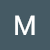# [Leet Code] Reverse Vowels of a String

Problem:

Given a string `s`, reverse only all the vowels in the string and return it.

The vowels are `'a'`, `'e'`, `'i'`, `'o'`, and `'u'`, and they can appear in both cases.

Example 1:

`Input: s = "hello"Output: "holle"`

Example 2:

`Input: s = "leetcode"Output: "leotcede"`

Solution:

`class Solution(object):    def reverseVowels(self, s):        """        :type s: str        :rtype: str        """        vowels = ["a", "e", "i", "o", "u", "A", "E", "I", "O", "U"]        if s == "":            return ""        s = list(s)        ending = len(s) -1        starting = 0        while starting < ending:            if s[starting] in vowels:                if s[ending] in vowels:                    temp = s[starting]                    s[starting] = s[ending]                    s[ending] = temp                    starting += 1                    ending -= 1                else:                    ending -= 1            elif s[ending] in vowels and s[starting] not in vowels:                starting +=1             else:                starting += 1                ending -= 1         return "".join(s)`

# [Leet Code] Find and Replace Pattern

Problem:

Given a list of strings `words` and a string `pattern`, return a list of `words[i]` that match `pattern`. You may return the answer in any order.

A word matches the pattern if there exists a permutation of letters `p` so that after replacing every letter `x` in the pattern with `p(x)`, we get the desired word.

Recall that a permutation of letters is a bijection from letters to letters: every letter maps to another letter, and no two letters map to the same letter.

Example 1:

`Input: words = ["abc","deq","mee","aqq","dkd","ccc"], pattern = "abb"Output: ["mee","aqq"]Explanation: "mee" matches…`

# [Leet Code] Reverse Prefix of Word

Problem:

Given a 0-indexed string `word` and a character `ch`, reverse the segment of `word` that starts at index `0` and ends at the index of the first occurrence of `ch` (inclusive). If the character `ch` does not exist in `word`, do nothing.

• For example, if `word = "abcdefd"` and `ch = "d"`, then you should reverse the segment that starts at `0` and ends at `3` (inclusive). The resulting string will be `"dcbaefd"`.

Return the resulting string.

Example 1:

`Input: word = "abcdefd", ch = "d"Output: "dcbaefd"Explanation: The first occurrence of "d" is at index 3…`

# [Leet Code] Build Array from Permutation

Problem:

Given a zero-based permutation `nums` (0-indexed), build an array `ans` of the same length where `ans[i] = nums[nums[i]]` for each `0 <= i < nums.length` and return it.

A zero-based permutation `nums` is an array of distinct integers from `0` to `nums.length - 1` (inclusive).

Example 1:

`Input: nums = [0,2,1,5,3,4]Output: [0,1,2,4,5,3]Explanation: The array ans is built as follows: ans = [nums[nums], nums[nums], nums[nums], nums[nums], nums[nums], nums[nums]]    = [nums, nums, nums, nums, nums, nums]    = [0,1,2,4,5,3]`

Example 2:

`Input: nums = [5,0,1,2,3,4]Output: [4,5,0,1,2,3]Explanation: The array ans is built as follows:ans = [nums[nums], nums[nums], nums[nums]…`

# [Leet Code] Length of Last Word

Problem

Given a string `s` consisting of some words separated by some number of spaces, return the length of the last word in the string.

A word is a maximal substring consisting of non-space characters only.

Example 1:

`Input: s = "Hello World"Output: 5Explanation: The last word is "World" with length 5.`

Example 2:

`Input: s = "   fly me   to   the moon  "Output: 4Explanation: The last word is "moon" with length 4.`

Example 3:

`Input: s = "luffy is still joyboy"Output: 6Explanation: The last word is "joyboy" with length 6.`## Matthew Boyd

Learning, and posting my findings!